Triangle eq

Calculate accurate to hundredths cm height of an equilateral triangle with a side length 12 cm. Calculate also its perimeter and content area.

Result

h =  10.39 cm
o =  36 cm
S =  62.35 cm2

Solution:Try calculation via our triangle calculator.

Leave us a comment of example and its solution (i.e. if it is still somewhat unclear...):Be the first to comment!To solve this example are needed these knowledge from mathematics:

See also our trigonometric triangle calculator. Pythagorean theorem is the base for the right triangle calculator.

Next similar examples:

1. Broken treeThe tree is broken at 4 meters above the ground and the top of the tree touches the ground at a distance of 5 from the trunk. Calculate the original height of the tree.
2. Areaf of ST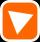It is given square DBLK with side |BL|=13. Calculate area of triangle DKU if vertex U lie on line LB.
3. RT 10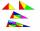Area of right triangle is 84 cm2 and one of its cathethus is a=10 cm. Calculate perimeter of the triangle ABC.
4. Right triangle ABCCalculate the perimeter and area of a right triangle ABC, if you know the length of legs 4 cm 5.5 cm and 6.8 cm is hypotenuse.
5. BaseCompute base of an isosceles triangle, with the arm a=20 cm and a height above the base h=10 cm.The ladder has a length 3.5 meters. He is leaning against the wall so that his bottom end is 2 meters away from the wall. Determine the height of the ladder.
7. Sides of triangle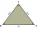Triangle circumference with two identical sides is 117cm. The third side measures 44cm. How many cms do you measure one of the same sides?
8. OPT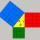What is the perimeter of a right triangle with the legs 14 cm and 21 cm long?
9. Center traverseIt is true that the middle traverse bisects the triangle?Ladder 6.4 meters long is positioned in the well such that its lower end is distanced from the wall of the well 1.2 m. The upper part of the ladder is supported on the upper edge of the well. How high is the well?8.3 meters long ladder is leaning against the wall of the well, and its lower end is 1.2 meters from this wall. How high from the bottom of a well is the top edge of the ladder?Construct a triangle ABC is is given c = 60mm hc = 40 mm and b = 48 mm analysis procedure steps construction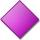Circumference of the rhombus is 44 cm, its height is 89 mm long. Calculate its content area.Calculate the area of a circle in dm2, if its circumference is 31.4 cm.Calculate the diameter and radius of the circle if it has length 52.45 cm.In the park there is a large circular flowerbed with a diameter of 12 m. Jakub circulated him ten times and the smaller Vojtoseven times. How many meters each went by and how many meters did Jakub run more than Vojta?Trapezium has an area of 24 square cms. How many different trapeziums can be formed ?